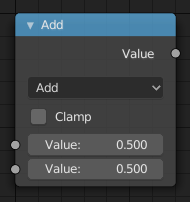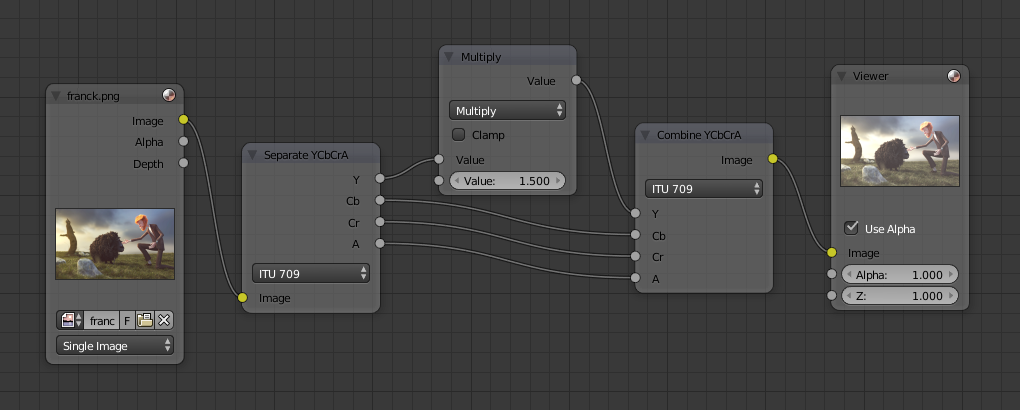# Math Node¶Math node.

The Math Node performs math operations.

## Inputs¶

Value
First numerical value. The trigonometric functions accept values in radians.
Value
Second numerical value. This value is not used in functions that accept only one parameter like the trigonometric functions, Round and Absolute.

## Properties¶

Operation
Add, Subtract, Multiply, Divide, Sine, Cosine, Tangent, Arcsine, Arccosine, Arctangent, Arctangent2, Power, Logarithm, Minimum, Maximum, Round, Less Than, Greater Than, Modulo, Absolute
Clamp
Limits the output to the range (0 to 1). See clamp.

## Outputs¶

Value
Numerical value output.

## Examples¶

### Manual Z-Mask¶Minimum and maximum function example.

This example has one scene input by the top Render Layers node, which has a cube that is about 10 units from the camera. The bottom Render Layers node inputs a scene with a plane that covers the left half of the view and is 7 units from the camera. Both are fed through their respective Map Value nodes to divide the Z-buffer by 20 (multiply by 0.05, as shown in the Size field) and clamped to be a min/max of 0.0/1.0 respectively.

For the minimum function, the node selects those Z values where the corresponding pixel is closer to the camera; so it chooses the Z values for the plane and part of the cube. The background has an infinite Z value, so it is clamped to 1.0 (shown as white). In the maximum example, the Z values of the cube are greater than the plane, so they are chosen for the left side, but the plane Render Layers Z are infinite (mapped to 1.0) for the right side, so they are chosen.

### Using Sine Function to Pulsate¶Using sine function example.

This example has a Time node putting out a linear sequence from 0 to 1 over the course of 101 frames. At frame 25, the output value is 0.25. That value is multiplied by 2 × pi (6.28) and converted to 1.0 by the Sine function, since we all know that $$sin(2 × pi/ 4) = sin(pi/ 2) = +1.0$$.

Since the sine function can put out values between (-1.0 to 1.0), the Map Value node scales that to 0.0 to 1.0 by taking the input (-1 to 1), adding 1 (making 0 to 2), and multiplying the result by one-half (thus scaling the output between 0 to 1). The default Color Ramp converts those values to a gray-scale. Thus, medium gray corresponds to a 0.0 output by the sine, black to -1.0, and white to 1.0. As you can see, $$sin(pi/ 2) = 1.0$$. Like having your own visual color calculator! Animating this node setup provides a smooth cyclic sequence through the range of grays.

Use this function to vary, for example, the alpha channel of an image to produce a fading in/out effect. Alter the Z channel to move a scene in/out of focus. Alter a color channel value to make a color “pulse”.

### Brightening (Scaling) a Channel¶Scaling a channel example.

This example has a Math (Multiply) node increasing the luminance channel (Y) of the image to make it brighter. Note that you should use a Map Value node with min() and max() enabled to clamp the output to valid values. With this approach, you could use a logarithmic function to make a high dynamic range image. For this particular example, there is also a Bright/Contrast node that might give simpler control over brightness.

### Restrict Color Selection (Posterization)¶Posterization example.

In this example, we restrict the color values to be one of the six values: 0, 0.2, 0.4, 0.6, 0.8, 1.

To split up a continuous range of values between 0 and 1 to certain set of values, the following function is used: $$round(x × n - 0.5) / (n - 1)$$, where “n” is the number of possible output values, and “x” is the input pixel color. Read more about this function.

To implement this function in Blender, consider the node setup above. We string the math nodes into a function that takes each color (values from 0 to 1), multiplies it up by six, the desired number of divisions (values become from 0 to 6), offsets it by 0.5 (-0.5 to 5.5), rounds the value to the nearest whole number (produces 0, 1, 2, 3, 4, 5), and then divides the image pixel color by five (0.0, 0.2, 0.4, 0.6, 0.8, 1.0).

In the case of a color image, you need split it into separate RGB channels using Separate/Combine RGBA nodes and perform this operation on each channel independently.